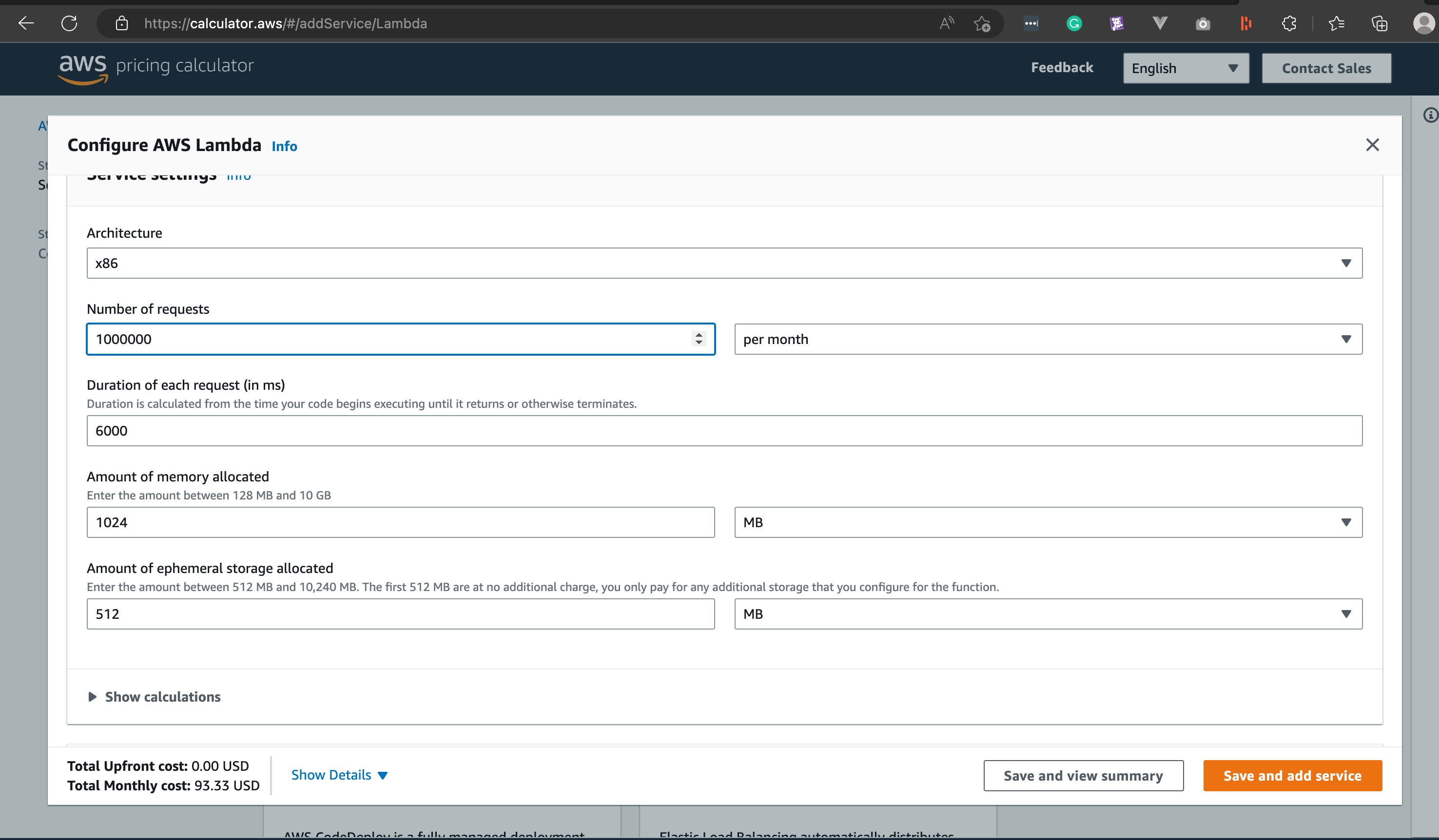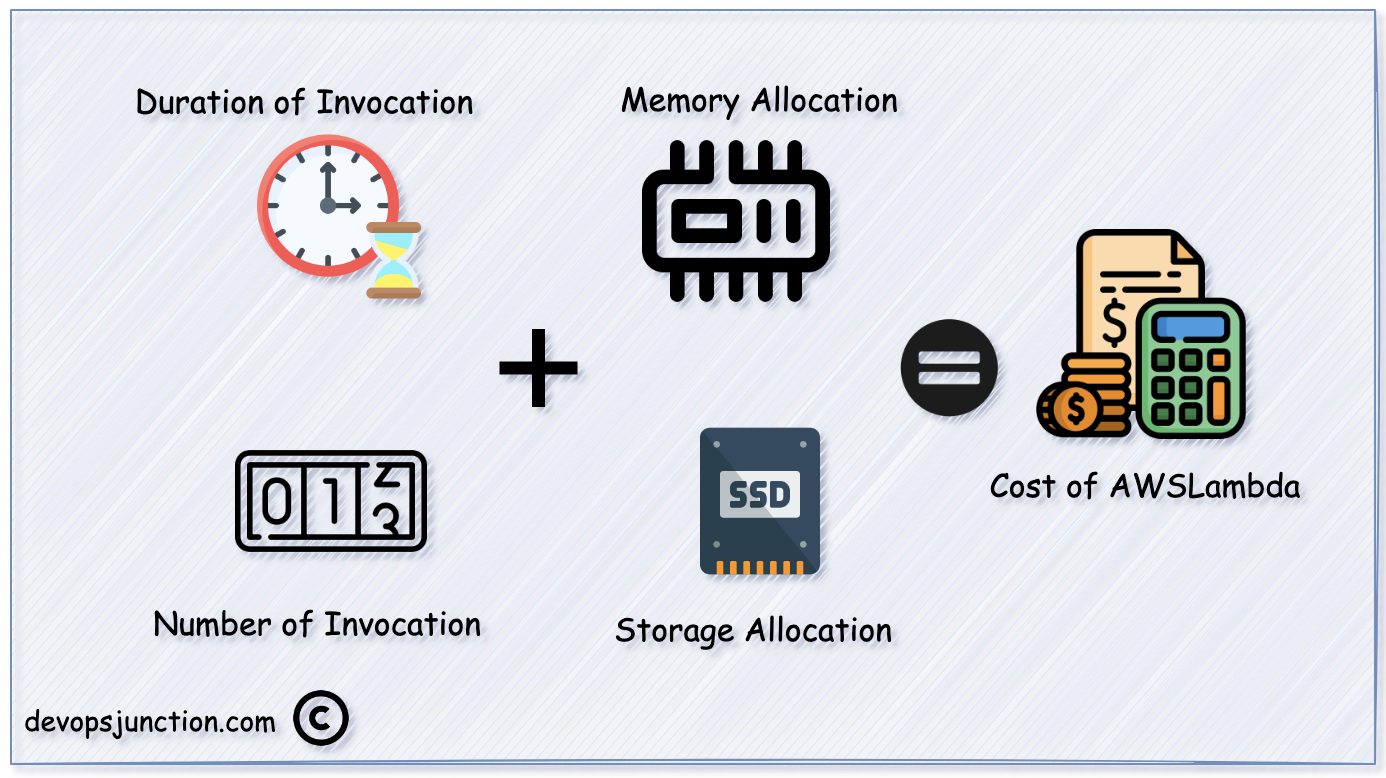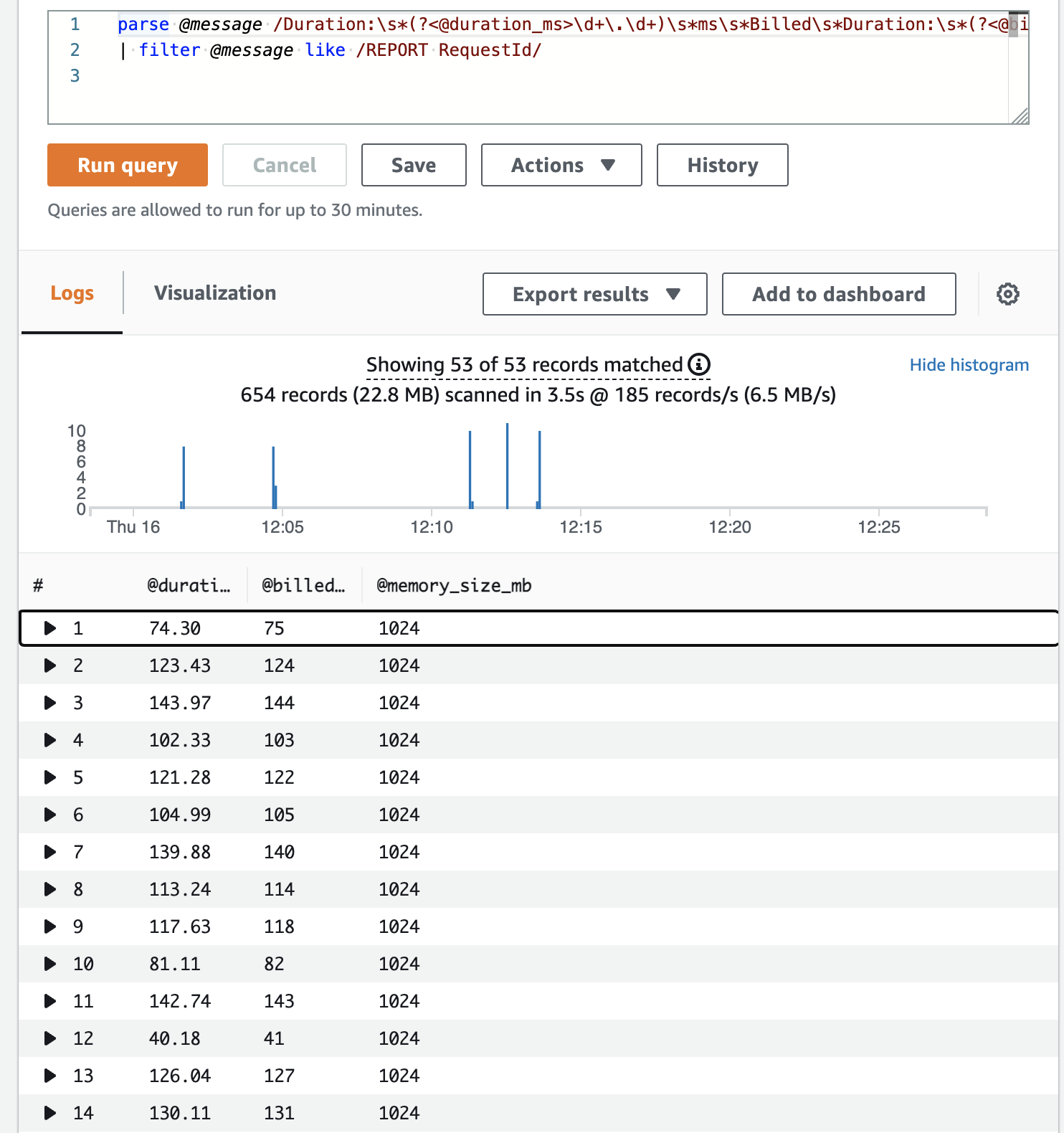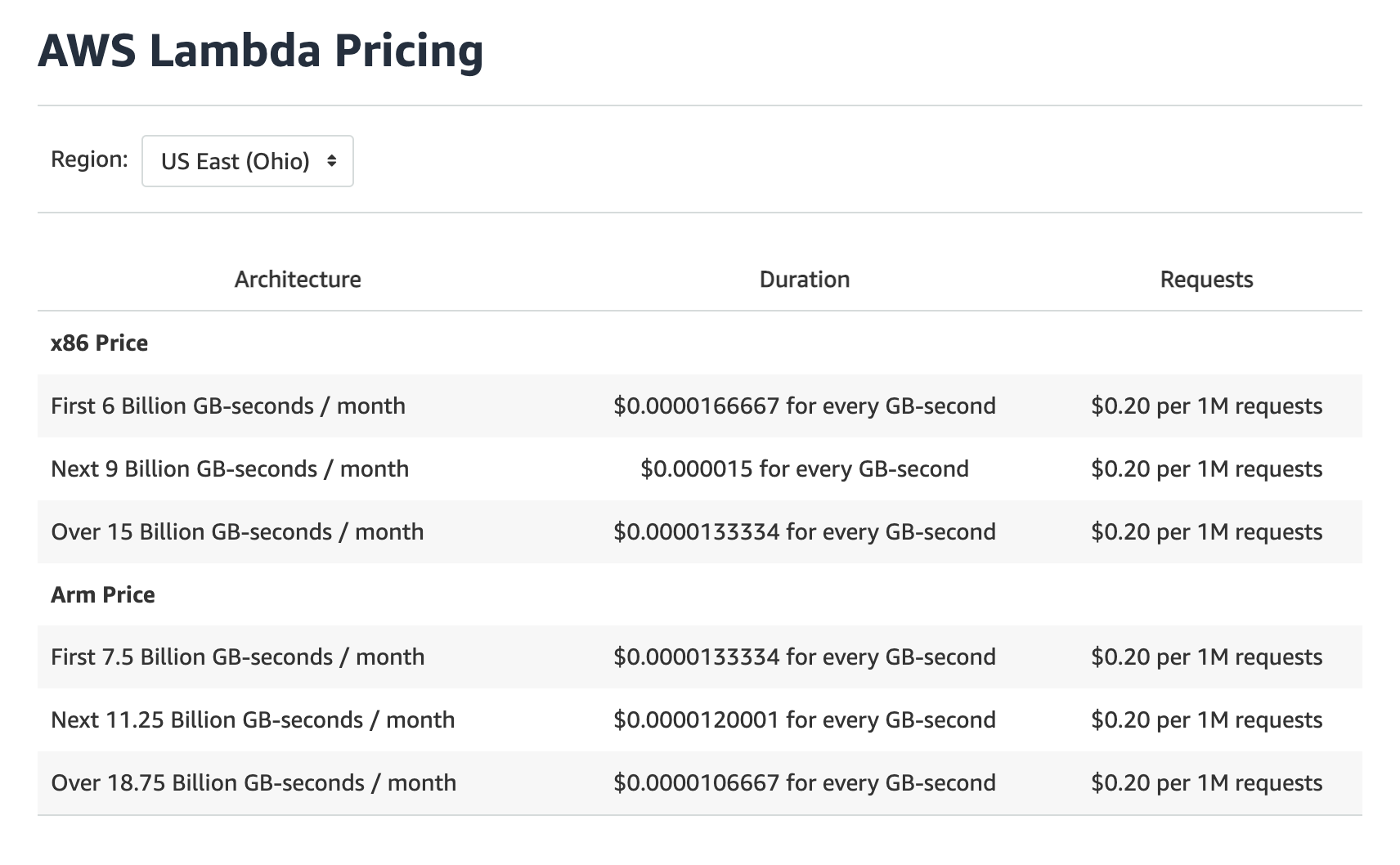# How to Calculate Cost per Lambda Function - AWS | Devops Junction

Serverless, as the name suggests takes the hosting and complex infrastructure elements away and helps developers and programmers to deploy their code to Production.

All the underlying infrastructure, availability, and scaling would be taken care of by the service provider.

For Cloud Functions its Google,  ForLambda its AWS so on and so forth

When we say they take care of the hosting and the infrastructure. We need to know how much they will charge for the same.

While most of the cloud providers give generous cost models, sometimes it is hard to calculate how much we are spending towards serverless.

In this article, let us talk about how AWS Lambda serverless cost is calculated and how we can calculate the cost per lambda function when there are too many.

## How to calculate AWS Lambda Costs?

If you do not want to dig too much deeper and simply try to create a quote or estimation, the right place is calculator.awsBut if you would like to understand a big Number in your bill or want to find out which Lambda function costs you how many dollars or how the Cost is calculated for Lamda.

## How AWS Lambda cost is calculated?

Four Key Elements that determine your aws lambda function cost

• Number of Requests for the Function
• Duration of each Request handled by the Function
• Allocated Memory for the Function
• Storage allocated to the Function## How to calculate the cost of Single Lambda?

You can use the following Cloud Watch Log Insight query to calculate the cost

```parse @message /Duration:\s*(?<@duration_ms>\d+\.\d+)\s*ms\s*Billed\s*Duration:\s*(?<@billed_duration_ms>\d+)\s*ms\s*Memory\s*Size:\s*(?<@memory_size_mb>\d+)\s*MB/
| filter @message like /REPORT RequestId/
| stats sum(@billed_duration_ms * @memory_size_mb * 1.6279296875e-11 + 2.0e-7) as @cost_dollars_total
```

Let's decode it

Every Single Lambda Function invocation log would contain a log similar to the following containing the following information

• Request ID
• Duration
• Billed Duration
• Memory Size
• Max Memory Used
```REPORT RequestId: 960e6de1-beb3-5234-adcd-318694aae239
Duration: 74.30 ms
Billed Duration: 75 ms
Memory Size: 1024 MB
Max Memory Used: 132 MB
```

We are basically filtering this log entry from the Lambda Function logs and Parse it to make some meaningful data.Finally, we are going to do the math with the following formula

`stats sum(@billed_duration_ms * @memory_size_mb * 1.6279296875e-11 + 2.0e-7) as @cost_dollars_total`

billed duration milliseconds multiplied by memory size in MB

further multiplied by `1.6279296875e-11 + 2.0e-7`

This is not a magic number, It is derived from the cost model and the numbers set by AWS itself

You can refer the following screen shot or directly from this pagethis Constant/Number `1.6279296875e-11` is derived from the following formula for the Duration

`\$0.00001667 for every GB-second / 1024 / 1000 = \$1.6279296875e-11 for every MB-millisecond.`

and the other number 2.0e-7 is an invocation cost, Calculated as follows

`0.20 / 1million = 2.0e-7`

that brings us to the our final calculation and validation and this is how the result would look like for a single Lambda## Conclusion

You can use the cloud watch insight query given to calculate the cost of lambda function. While we only focus on only the Duration and the invocation there might be a slight addition to this cost based on the storage allocated.

You can also use cost explorer to get the overall Lambda Usage

Hope this article helps you understand the billing model and the cost calculation logic of Lambda.

Feel free to share if you have any better options or ideas to get this done.